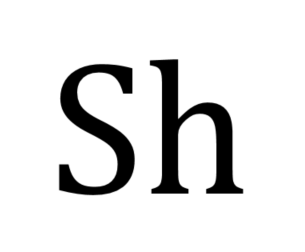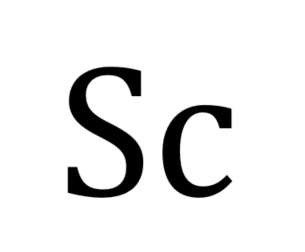f
Neutrium

# Mass Transfer

Sherwood NumberThe Sherwood number is a dimensionless number that represents the ratio of convective mass transfer to the rate of diffusive mass transport and is used in the analysis of mass transfer systems such as liquid-liquid extraction. This article describes the Sherwood number and typical formulations.

Schmidt NumberThe Schmidt number is a dimensionless number that describes the ratio of momentum diffusivity to mass diffusivity that is commonly used in analysis of mass transfer systems. This article describes the Schmidt Number and typical formulations.